# 9) A gas of initial volume 2.50 x 10-3 m3 does 684 J of work on...

9) A gas of initial volume 2.50 x 10-3 m3 does 684 J of work on its surrounding. During this process the gas pressure was maintained at 1.70 x 105Pa. Find the final volume of the gas.

3.11 x 10-3 m3

6.52 x 10-3 m3

4.02 x 10-3 m3

12.7 x 10-3 m3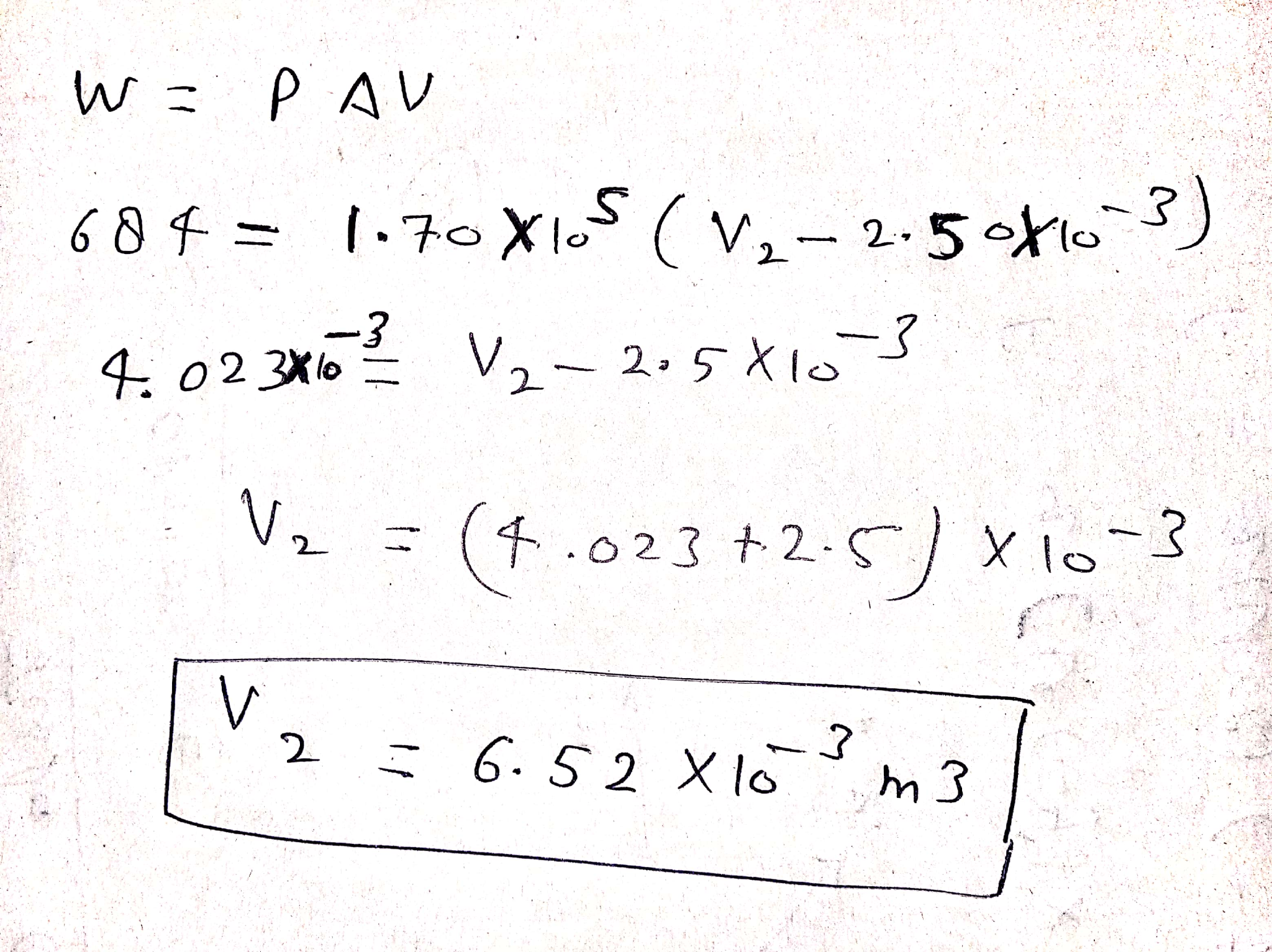#### Earn Coin

Coins can be redeemed for fabulous gifts.

Similar Homework Help Questions
• ### An ideal monatomic gas is contained in a vessel of constant volume 0.470 m3. The initial...

An ideal monatomic gas is contained in a vessel of constant volume 0.470 m3. The initial temperature and pressure of the gas are 300 K and 5.00 atm, respectively. The goal of this problem is to find the temperature and pressure of the gas after 30.0 kJ of thermal energy is supplied to the gas. (a) Use the ideal gas law and initial conditions to calculate the number of moles of gas in the vessel. mol (b) Find the specific...

• ### An ideal gas is brought through an isothermal compression process. The 5.00 mol of gas goes...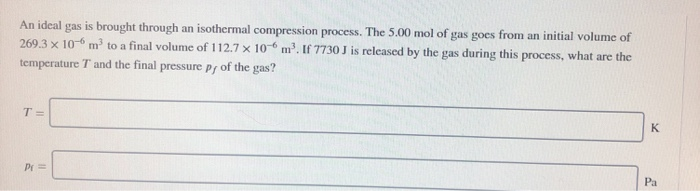An ideal gas is brought through an isothermal compression process. The 5.00 mol of gas goes from an initial volume of 269 3 10-6 rm3 to a final volume of 1 12.7 10 6 m, If 7730 J İs released by the gas during this process, what are the temperature T and the final pressure py of the gas? Pa

• ### A0.01 m3 container holds a particular gas at an initial temperature of 283 K. The container...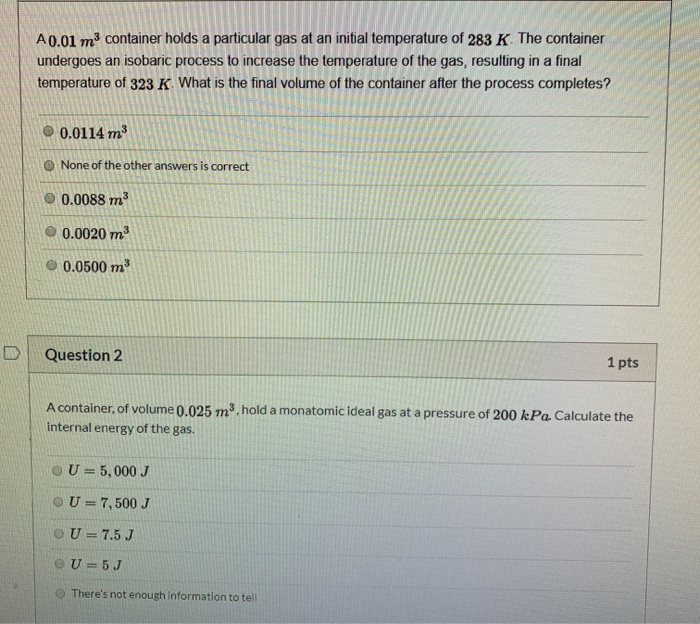A0.01 m3 container holds a particular gas at an initial temperature of 283 K. The container undergoes an isobaric process to increase the temperature of the gas, resulting in a final temperature of 323 K What is the final volume of the container after the process completes? 0.0114 m None of the other answers is correct 0.0088 m3 0.0020 m3 0.0500 m Question 2 1 pts A container, of volume 0.025 m3 hold a monatomic ideal gas at a pressure...

• ### 105Pa, initial temperature T-300K, and an initial 1. An ideal gas with initial pressure 2 volume V - 1m3 expands isothermally to a final volume of 2m3. Then, the gas returns to its initial state, fir...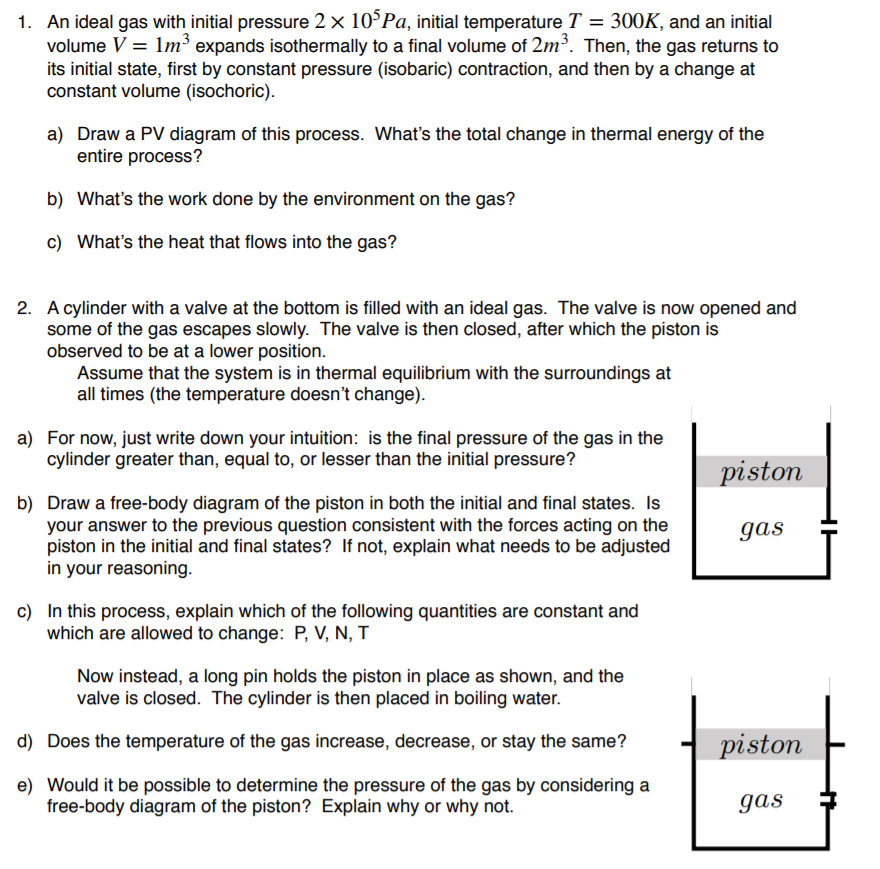105Pa, initial temperature T-300K, and an initial 1. An ideal gas with initial pressure 2 volume V - 1m3 expands isothermally to a final volume of 2m3. Then, the gas returns to its initial state, first by constant pressure (isobaric) contraction, and then by a change at constant volume (isochoric) a) Draw a PV diagram of this process. What's the total change in thermal energy of the entire process? b) What's the work done by the environment on the gas?...

• ### phpnd 6874594 Bryson Grant ate University P255-Spring19- CASH Activities and Due Dates HW 3: Ideal Gas...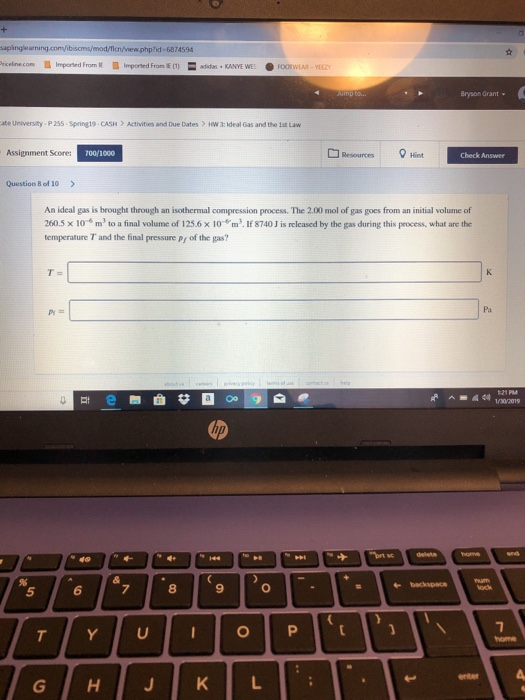phpnd 6874594 Bryson Grant ate University P255-Spring19- CASH Activities and Due Dates HW 3: Ideal Gas and the 1st Law Assignment Scores 00/1000 Resources 8+int Check Answer Question 8 of 10> An ideal gas is brought through an isothermal compression process. The 2.00 mol of gas goes from an initial volume of 260.5 x 10-s m2 to a final volume of 125.6 x 10 m3. If 8740 J is released by the gas during this process, what are the temperature...

• ### A volume of air (assumed to be an ideal gas) is first cooled without changing its...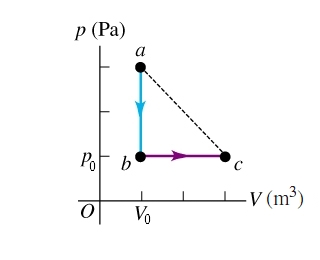A volume of air (assumed to be an ideal gas) is first cooled without changing its volume and then expanded without changing its pressure, as shown by the path abc in the figure (Figure 1). Take the graduation p0=2.5×105Pa and the graduation V0=0.05. How does the final temperature of the gas compare with its initial temperature? How much heat does the air exchange with its surroundings during the process abc? If the air instead expands from state a to state...

• ### 4-10 A gas is compressed from an initial volume of 0.42 m^3 to a final volume...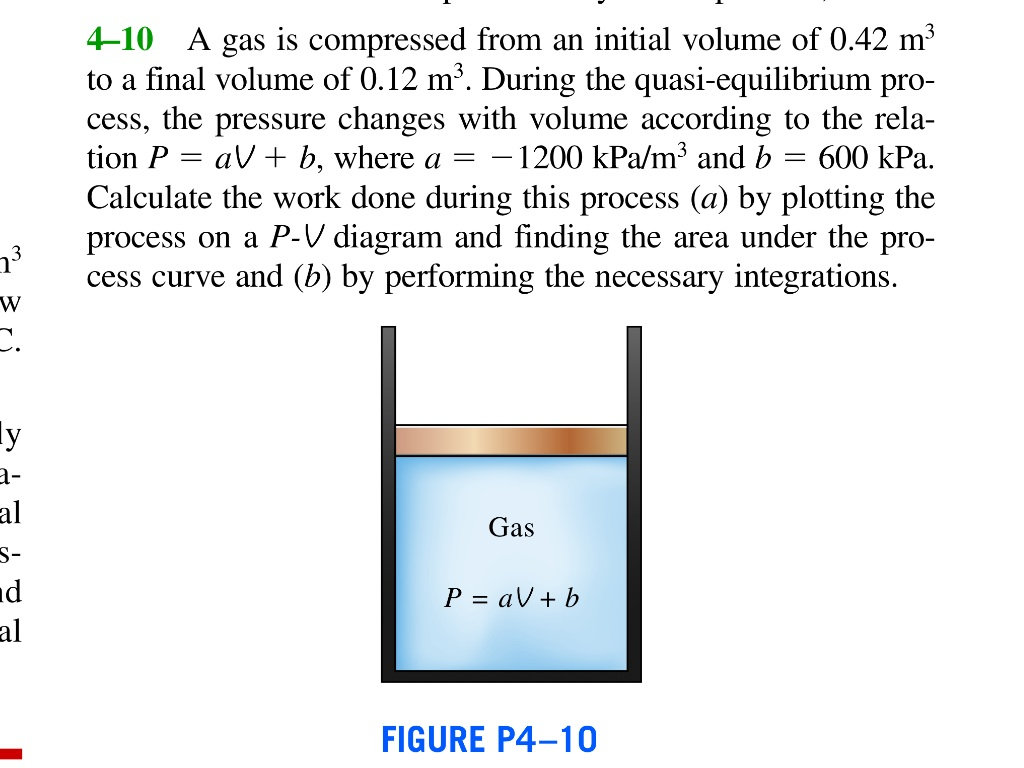4-10 A gas is compressed from an initial volume of 0.42 m^3 to a final volume of 0.12 mm. During the quasi-equilibrium process, the pressure changes with volume according to the relation P = aV + b, where a = -1200 kPa/m^3 and b = 600 kPa. Calculate the work done during this process (a) by plotting the process on a P-V diagram and finding the area under the process curve and (b) by performing the necessary integrations.

• ### Ideal Gas: Please show all work and explain (a) An ideal gas expands adiabatically from a...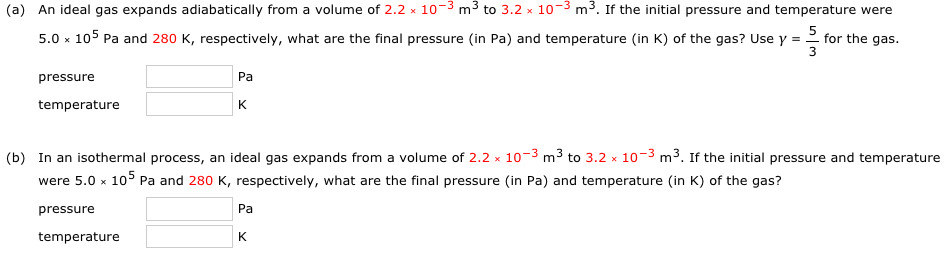Ideal Gas: Please show all work and explain (a) An ideal gas expands adiabatically from a volume of 2.2 × 10-3 m3 to 3.2 × 10-3 m3. If the initial pressure and temperature were 5 pressure Pa temperature (b) In an isothermal process, an ideal gas expands from a volume of 2.2 10-3 m3 to 3.2 10-3 m3. If the initial pressure and temperature were 5.0 x 105 Pa and 280 K, respectively, what are the final pressure (in Pa)...

• ### The work done on an ideal gas is -5.41 x 10^6 J during a process in...

The work done on an ideal gas is -5.41 x 10^6 J during a process in which the temperature remains constant at 323 C and the volume changes from 2.14 m3 to 7.28 m3? How many moles of the gas are there? Express your result to three significant digits and in scientific notation. Be careful with signs!

• ### Question 1) A gas is compressed from an initial volume of 0.42 mº to a final...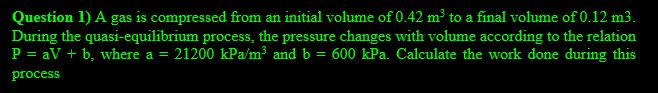Question 1) A gas is compressed from an initial volume of 0.42 mº to a final volume of 0.12 m3. During the quasi-equilibrium process, the pressure changes with volume according to the relation P = aV + b, where a = 21200 kPa/m2 and b = 600 kPa. Calculate the work done during this process